Setting the Starting Page Number

This post will guide you how to set the starting page number in the header of footer in Excel. How do I set the starting page number at 2 or other different number in excel. How to change the starting page number in excel 2013/2016?

You should know that the default starting page number is number 1. Assuming that you have a cover page for your excel file, and you may be want to change the starting number at number 2, and how to achieve this result. You just need to do the following steps:

You can follow these steps to insert page number and then set the starting number at 2 or any different number in your current worksheet.

#1 go to INSERT tab, click Header&Footer command under Text group.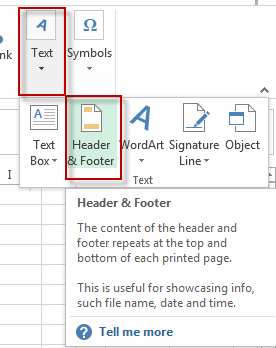#2 go to DESIGN tab, click Page Number command under Header&Footer Elements group. Then you will see that the page number has been inserted in your worksheet.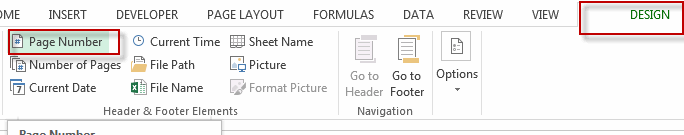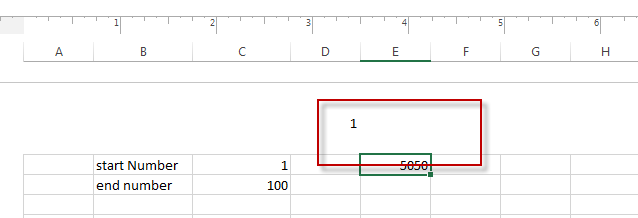#3 go to PAGE LAYOUT tab, click the anchor at the bottom-right corner of the Page Setup group. The Page Setup dialog will appear.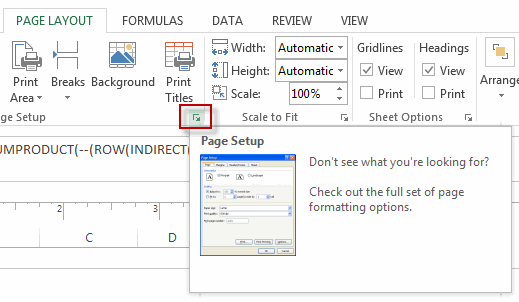#4 switch to Page tab, type number 2 into the box of First page number or other numbers that you want to set the starting page number.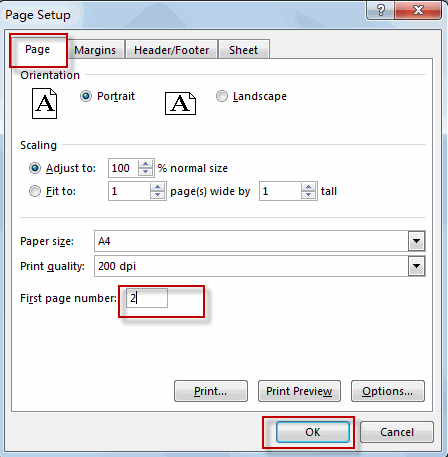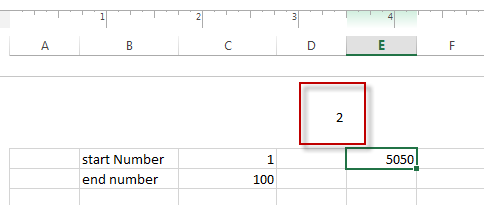So far, the starting page number has been set as number 2.

Related Posts

Filter Data by Column and Sort by Row

This post will guide you how to use the FILTER function to filter a value by column and then sort data by row in Microsoft Excel. You can use the following formula based on the SORT function in combination with ...

Filter And Transpose Data From Horizontal To Vertical

This post will show you how to use Filter function and in combination with Transpose function to filter data from horizontal and transpose data as vertical in Microsoft Excel. You can refer to the below general formula based on TRANSPOSE ...

VLOOKUP Formula | Faster Trick with 2 VLOOKUPS

This post will guide you how to use 2 VLOOKUPS function to looking up data entries from a given range of cells in Microsoft Excel. VLOOKUP with 2 lookups can be faster than a single VLOOKUP in certain scenarios. The ...

Extract Unique Items From A List

This post will guide you how to extract unique itmes from a given list in Microsoft Excel. How to create a newly formula to get unique values from a range cells in Excel. The unique list of items is the ...

Extract substring In Excel

This post will guide you how to use Excel's MID function is a quick and easy way to extract pieces from your text. Use the Excel formula to extract a substring with MID. Note: If you want to extract just ...

How to Use 3D SUM Multiple Worksheets

To sum a range of numbers is straightforward for most Excel users, but do you know how to establish a 3D reference to total the same range of numerous sheets. In this post, I will present the steps for this ...

Extract all the matches with helper Column

With Excel's powerful functions IF, INDEX, and MATCH, we can find exactly what you're looking for with a few clicks of the mouse. This step-by-step tutorial will show how easy it is to extract data using these tools and more! ...

If Cell is This Value or That Value

IF function is frequently used in Excel worksheet to return you expect “true value” or “false value” based on the result of logical test. If you want to see if a cell is A or B, and if one of ...

If Value is Greater Than A Certain Value

IF function is frequently used in Excel worksheet to return you expect “true value” or “false value” based on the logical test result. If you want to see if a value in one cell is greater than a specific value, ...

If Cell is Not Blank

IF function is frequently used in Excel worksheet to return you expect “true value” or “false value” based on the result of created logical test. If you want to see if a cell is blank or not, and leave some ...

Sidebar